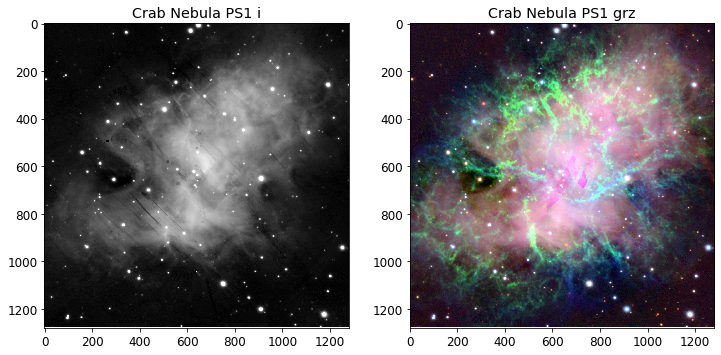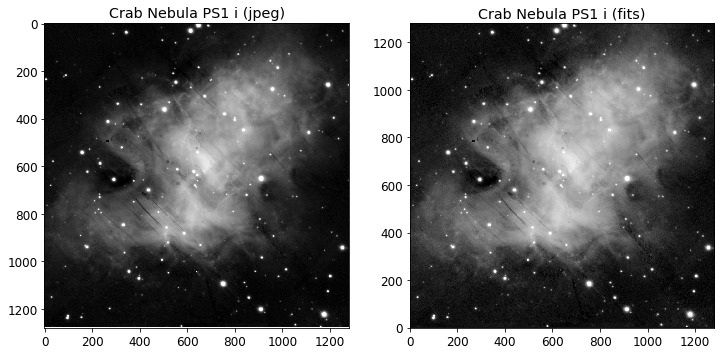# Get image from the PS1 image server¶

Query the PS1 image server to get a list of images and retrieve some images. This sample script demonstrates the use of the PS1 image services. See the PS1 Image Cutout Service documentation for details of the services being used. This notebook is available for download.

In :
import numpy
from astropy.table import Table
import requests
from PIL import Image
from io import BytesIO
import matplotlib.pyplot as plt


## Helper functions to query the list of images and to extract images¶

In :
def getimages(ra,dec,filters="grizy"):

"""Query ps1filenames.py service to get a list of images

ra, dec = position in degrees
size = image size in pixels (0.25 arcsec/pixel)
filters = string with filters to include
Returns a table with the results
"""

service = "https://ps1images.stsci.edu/cgi-bin/ps1filenames.py"
url = f"{service}?ra={ra}&dec={dec}&filters={filters}"
return table

def geturl(ra, dec, size=240, output_size=None, filters="grizy", format="jpg", color=False):

"""Get URL for images in the table

ra, dec = position in degrees
size = extracted image size in pixels (0.25 arcsec/pixel)
output_size = output (display) image size in pixels (default = size).
output_size has no effect for fits format images.
filters = string with filters to include
format = data format (options are "jpg", "png" or "fits")
color = if True, creates a color image (only for jpg or png format).
Default is return a list of URLs for single-filter grayscale images.
Returns a string with the URL
"""

if color and format == "fits":
raise ValueError("color images are available only for jpg or png formats")
if format not in ("jpg","png","fits"):
raise ValueError("format must be one of jpg, png, fits")
table = getimages(ra,dec,filters=filters)
url = (f"https://ps1images.stsci.edu/cgi-bin/fitscut.cgi?"
f"ra={ra}&dec={dec}&size={size}&format={format}")
if output_size:
url = url + "&output_size={}".format(output_size)
# sort filters from red to blue
flist = ["yzirg".find(x) for x in table['filter']]
table = table[numpy.argsort(flist)]
if color:
if len(table) > 3:
# pick 3 filters
table = table[[0,len(table)//2,len(table)-1]]
for i, param in enumerate(["red","green","blue"]):
url = url + "&{}={}".format(param,table['filename'][i])
else:
urlbase = url + "&red="
url = []
for filename in table['filename']:
url.append(urlbase+filename)
return url

def getcolorim(ra, dec, size=240, output_size=None, filters="grizy", format="jpg"):

"""Get color image at a sky position

ra, dec = position in degrees
size = extracted image size in pixels (0.25 arcsec/pixel)
output_size = output (display) image size in pixels (default = size).
output_size has no effect for fits format images.
filters = string with filters to include
format = data format (options are "jpg", "png")
Returns the image
"""

if format not in ("jpg","png"):
raise ValueError("format must be jpg or png")
url = geturl(ra,dec,size=size,filters=filters,output_size=output_size,format=format,color=True)
r = requests.get(url)
im = Image.open(BytesIO(r.content))
return im

def getgrayim(ra, dec, size=240, output_size=None, filter="g", format="jpg"):

"""Get grayscale image at a sky position

ra, dec = position in degrees
size = extracted image size in pixels (0.25 arcsec/pixel)
output_size = output (display) image size in pixels (default = size).
output_size has no effect for fits format images.
filter = string with filter to extract (one of grizy)
format = data format (options are "jpg", "png")
Returns the image
"""

if format not in ("jpg","png"):
raise ValueError("format must be jpg or png")
if filter not in list("grizy"):
raise ValueError("filter must be one of grizy")
url = geturl(ra,dec,size=size,filters=filter,output_size=output_size,format=format)
r = requests.get(url)
im = Image.open(BytesIO(r.content))
return im


## Sample JPEG images¶

This gets single-band grayscale and color JPEG images at the position of the Crab Nebula. The extracted region size is 1280 pixels = 320 arcsec.

In :
# Crab Nebula position
ra = 83.633210
dec = 22.014460
size = 1280

# grayscale image
gim = getgrayim(ra,dec,size=size,filter="i")
# color image
cim = getcolorim(ra,dec,size=size,filters="grz")

plt.rcParams.update({'font.size':12})
plt.figure(1,(12,6))
plt.subplot(121)
plt.imshow(gim,origin="upper",cmap="gray")
plt.title('Crab Nebula PS1 i')
plt.subplot(122)
plt.title('Crab Nebula PS1 grz')
plt.imshow(cim,origin="upper")

Out:
<matplotlib.image.AxesImage at 0x7f8830317750>## Load and display a FITS image¶

Note that the $y$-axis is flipped in the JPEG image compared with the original FITS image.

In :
from astropy.io import fits
from astropy.visualization import PercentileInterval, AsinhStretch

fitsurl = geturl(ra, dec, size=size, filters="i", format="fits")
fh = fits.open(fitsurl)
fim = fh.data
# replace NaN values with zero for display
fim[numpy.isnan(fim)] = 0.0
# set contrast to something reasonable
transform = AsinhStretch() + PercentileInterval(99.5)
bfim = transform(fim)

plt.figure(1,(12,6))
plt.subplot(121)
plt.imshow(gim,cmap="gray",origin="upper")
plt.title('Crab Nebula PS1 i (jpeg)')

plt.subplot(122)
plt.title('Crab Nebula PS1 i (fits)')
plt.imshow(bfim,cmap="gray",origin="lower")

Out:
<matplotlib.image.AxesImage at 0x7f8810474110>In [ ]: